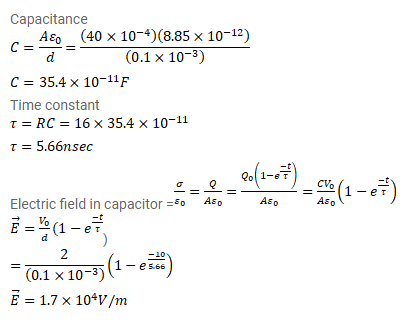# A parallel-plate capacitor has plate area

Question:

A parallel-plate capacitor has plate area $40 \mathrm{~cm}^{2}$ and separation between the plates $0.10 \mathrm{~mm}$ is connected to a battery of emf $2.0 \mathrm{~V}$ through a $16 \Omega$ resistor. Find the electric field in the capacitor $10 \mathrm{~ns}$ after the connections are made.

Solution: